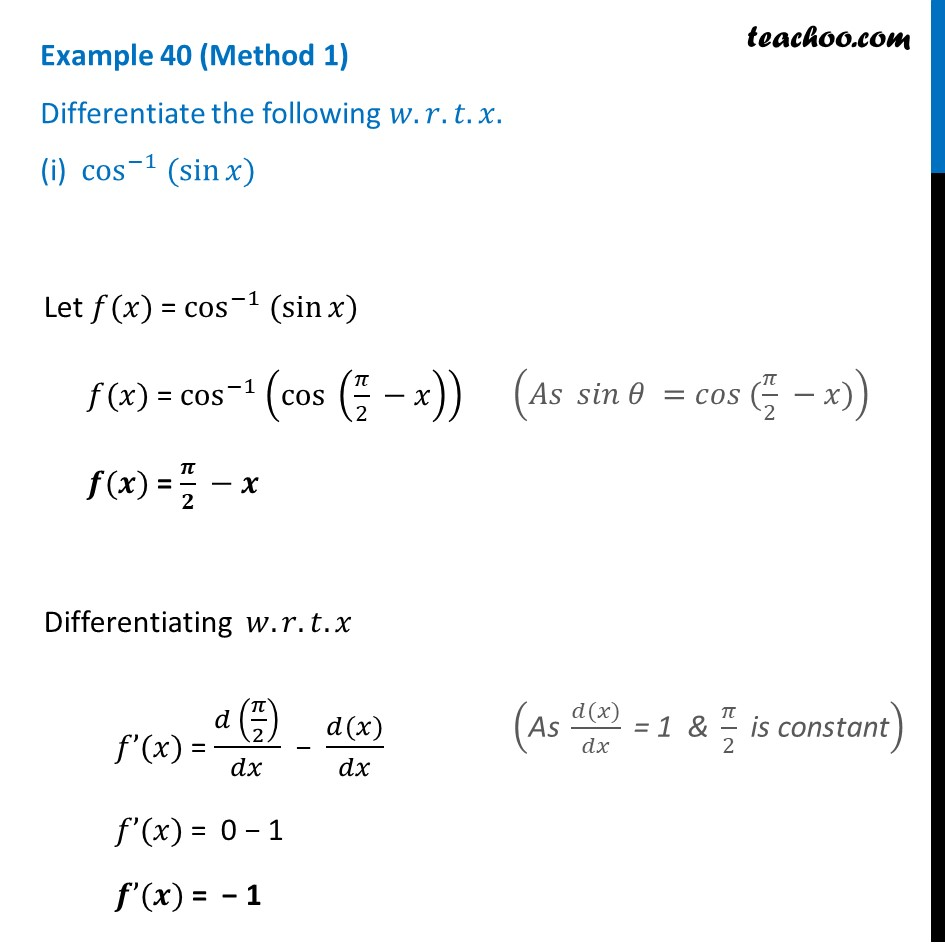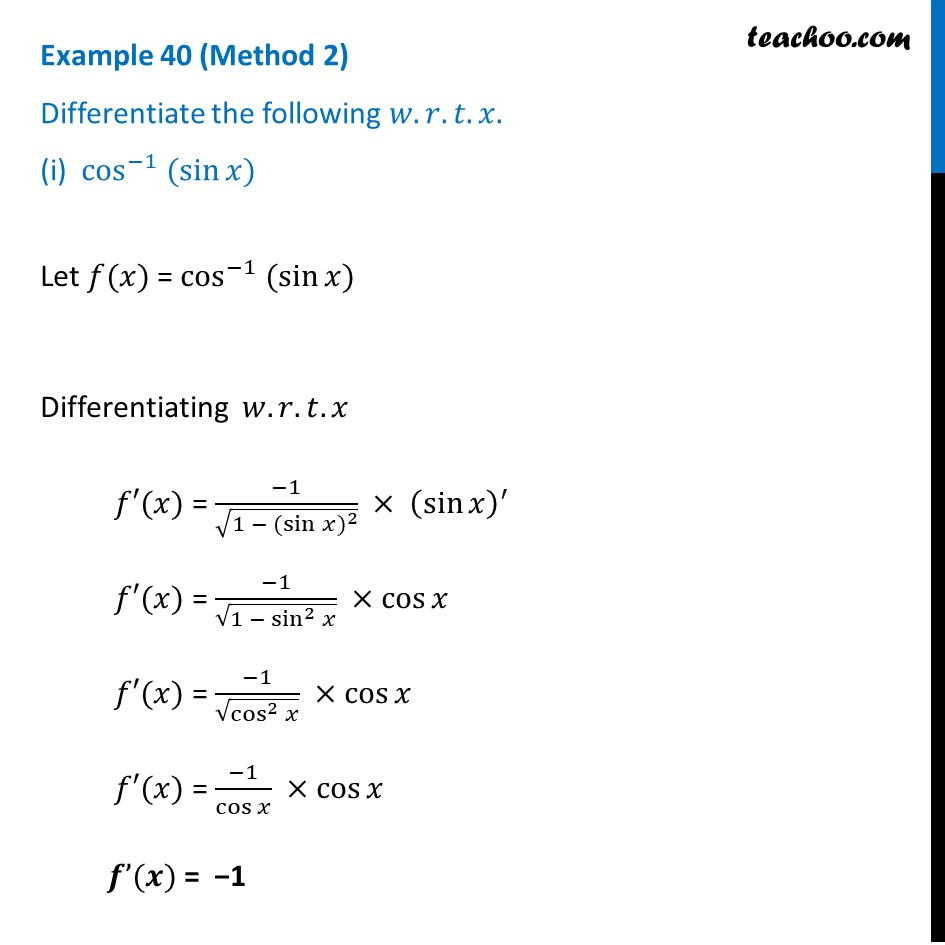Chapter 5 Class 12 Continuity and Differentiability

Class 12
Important Questions for exams Class 12Learn in your speed, with individual attention - Teachoo Maths 1-on-1 Class

### Transcript

Example 40 (Method 1) Differentiate the following 𝑤.𝑟.𝑡. 𝑥. (i) cos^(−1) (sin⁡𝑥) Let 𝑓(𝑥) = cos^(−1) (sin⁡𝑥) 𝑓(𝑥) = cos^(−1) (〖cos 〗⁡(𝜋/2 −𝑥) ) 𝒇(𝒙) = 𝝅/𝟐 −𝒙 Differentiating 𝑤.𝑟.𝑡.𝑥 𝑓’(𝑥) = (𝑑 (𝜋/2))/𝑑𝑥 − (𝑑(𝑥))/𝑑𝑥 𝑓’(𝑥) = 0 − 1 𝒇’(𝒙) = − 1(𝐴𝑠 〖 𝑠𝑖𝑛 𝜃 〗⁡〖=〖𝑐𝑜𝑠 〗⁡〖(𝜋/2 −𝑥)〗 〗 ) ("As " (𝑑(𝑥))/𝑑𝑥 " = 1 & " 𝜋/2 " is constant" ) Example 40 (Method 2) Differentiate the following 𝑤.𝑟.𝑡. 𝑥. (i) cos^(−1) (sin⁡𝑥) Let 𝑓(𝑥) = cos^(−1) (sin⁡𝑥) Differentiating 𝑤.𝑟.𝑡.𝑥 𝑓′(𝑥) = (−1)/√(1 − 〖(sin⁡𝑥)〗^2 ) × (sin⁡𝑥 )^′ 𝑓′(𝑥) = (−1)/√(1 − sin^2⁡𝑥 ) ×cos⁡𝑥 𝑓′(𝑥) = (−1)/√(cos^2⁡𝑥 ) ×cos⁡𝑥 𝑓′(𝑥) = (−1)/cos⁡𝑥 ×cos⁡𝑥 𝒇’(𝒙) = −1